×#### Thank you for registering.

One of our academic counsellors will contact you within 1 working day.

Click to Chat

1800-1023-196

+91-120-4616500

CART 0

• 0

MY CART (5)

Use Coupon: CART20 and get 20% off on all online Study Material

ITEM
DETAILS
MRP
DISCOUNT
FINAL PRICE
Total Price: Rs.

There are no items in this cart.
Continue Shopping
```
A particle of mass m is subjected to a net force (t) given by (t) that is, F(t) equals Fo at t = 0 and decreases linearly to zero in time T. The particle passes the origin x = 0 with velocity . Show that at the instant t = T that F(t) vanishes, the speed v and distance x traveled are given by v(T) and x(T) , where is the initial acceleration. Compare these results with Eqs. 2-26 and 2-28.
A particle of mass m is subjected to a net force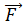(t) given by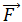(t)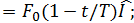that is, F(t) equals Fo at t = 0 and decreases linearly to zero in time T. The particle passes the origin x = 0 with velocity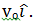. Show that at the instant t = T that F(t) vanishes, the speed v and distance x traveled are given by v(T)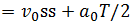and x(T)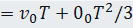, where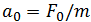is the initial acceleration. Compare these results with Eqs. 2-26 and 2-28.

```
5 years ago

```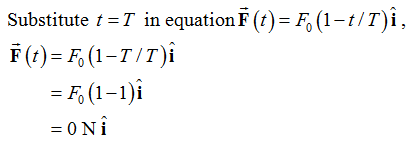Therefore the force experienced by the particle at t = T is zero .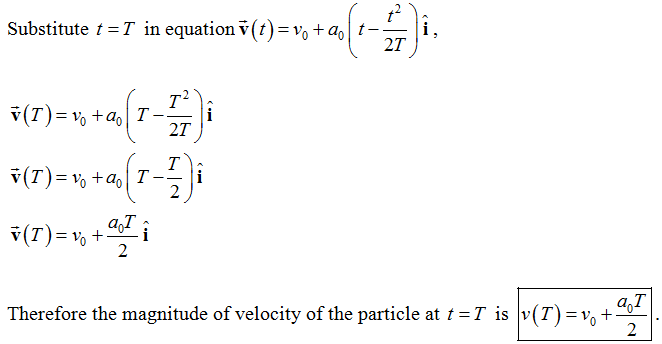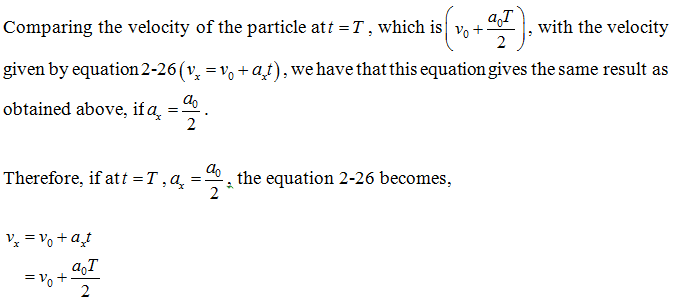Therefore, the velocity matches the magnitude of velocity calculated above.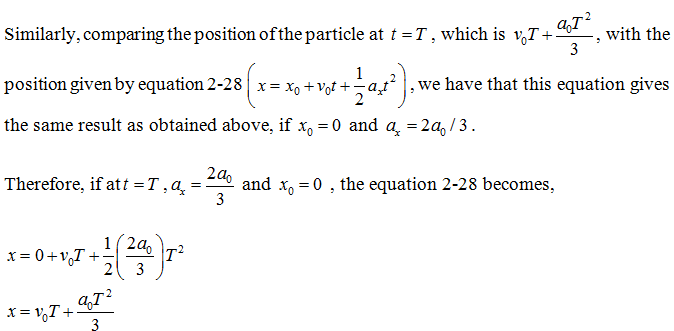Therefore, the position matches the magnitude of position calculated above
```
5 years ago
Think You Can Provide A Better Answer ?

## Other Related Questions on Mechanics

View all Questions »### Course Features

• 101 Video Lectures
• Revision Notes
• Previous Year Papers
• Mind Map
• Study Planner
• NCERT Solutions
• Discussion Forum
• Test paper with Video Solution### Course Features

• 110 Video Lectures
• Revision Notes
• Test paper with Video Solution
• Mind Map
• Study Planner
• NCERT Solutions
• Discussion Forum
• Previous Year Exam Questions